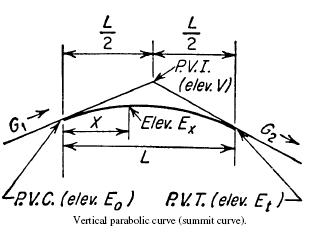Search

# Parabolic Curves

Posted in Highway Engineering |Email This Post |

Parabolic curves find there usage when we have to connect sections of highways or railroads of differing gradient. The use of a parabolic curve provides a gradual change in direction along the curve.where
PVC= point of vertical curvature, beginning of curve
PVI= point of vertical intersection of grades on either side of curve
PVT= point of vertical tangency, end of curve
G1= grade at beginning of curve, ft/ft (m/m)
G2= grade at end of curve, ft/ft (m/m)
L=length of curve, ft (m)
R =rate of change of grade, ft/ft2 (m/m2)
V =elevation of PVI, ft (m)

Eo= elevation of PVC, ft (m)
Et= elevation of PVT, ft (m)
x =distance of any point on the curve from the
PVC, ft (m)
Ex= elevation of point x distant from PVC, ft (m)
xs= distance from PVC to lowest point on a
sag curve or highest point on a summit curve, ft (m)
Es= elevation of lowest point on a sag curve or highest point on a summit curve, ft (m)

Equations of Parabolic Curves

R=(G2-G1)/L

Eo=V-LG1/2

Ex=Eo+G1x +Rx2/2

xs1/R

Eso-G12/2R

For xs>L:The curve has no high or low point